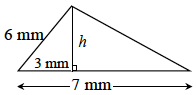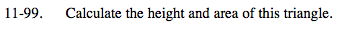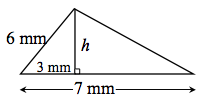Home > MC2 > Chapter 11 > Lesson 11.3.2 > Problem11-99

11-99.

Calculate the height and area of this triangle. Homework Help ✎Notice that there are two right triangles within this larger triangle.

For the smaller triangle, one leg and the hypotenuse have
known lengths. How can you use this information to find the
length of the other leg, and therefore, the height?

Use the Pythagorean Theorem.
a2 + b2 = c2

32 + h2 = 62
Solve for h.

$h = \sqrt{27} \approx 5.2 mm$

How can you use the information you have now to find the area?

$Area = \frac{1}{2}(base)(height)$